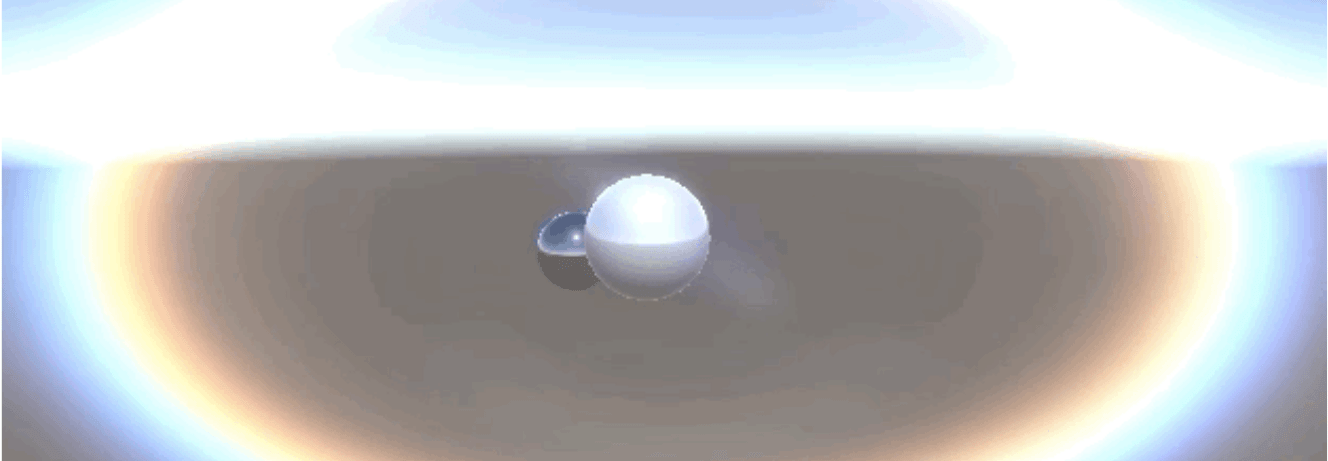# Unity镜头光晕模拟开源库

## 效果展示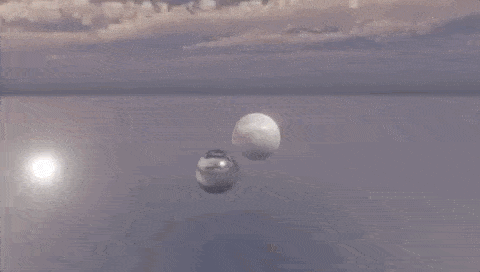## 效果实现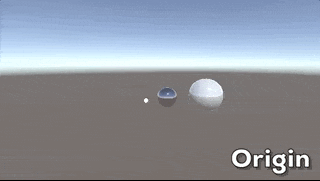1、Downsampling/Threshold

``````fixed4 frag (v2f i) : SV_Target
{
fixed4 col = tex2D(_MainTex, i.uv);
col = max(col-_Sub, 0);
col *= _Mul;
return col;
}
``````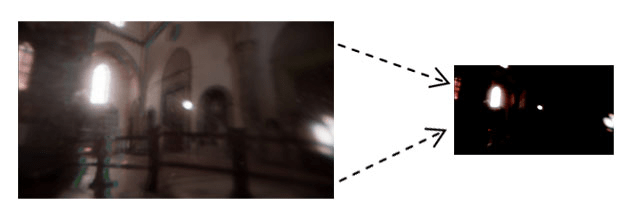2、Ghosts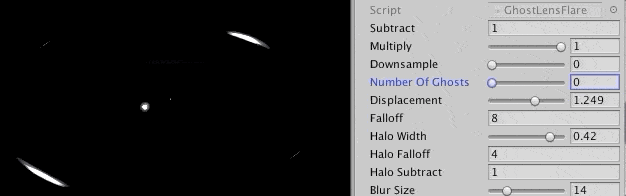``````fixed4 frag(v2f i) : SV_Target
{
fixed4 col = tex2D(_MainTex, i.uv);
float2 uv = i.uv - float2(0.5, 0.5);
for (int k = 3; k < _NumGhost + 3; k++) {
if (k & 1) {
col += tex2D(_MainTex, _Displace * -uv / (k >> 1) + float2(0.5, 0.5));
}
else {
col += tex2D(_MainTex, uv / (k >> 1) + float2(0.5, 0.5));
}
}
col *= pow(1 - length(uv) / .707, _Falloff);
return col;
}
``````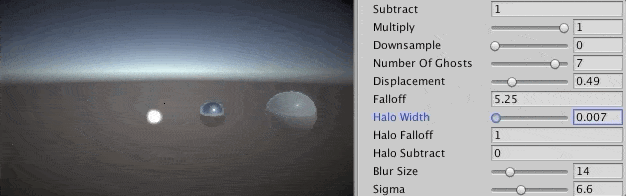``````fixed4 frag(v2f i) : SV_Target
{
fixed4 col = fixed4(0,0,0,0);

float2 ghostVec = i.uv - .5;
//归一化后减光环半径得到光环顶点位置
float2 haloVec = normalize(ghostVec)*-_HaloWidth;
//根据光环位置得到每个像素的权重，为一个白色的圆环
float weight = length(float2(0.5, 0.5) - (i.uv + haloVec)) / .707;
//添加衰减效果
weight = pow(1.0 - weight, _HaloFalloff);
col += tex2D(_MainTex, i.uv + haloVec) * weight;
col = max(0, col - _HaloSub);
return col;
}
``````

4、Aberration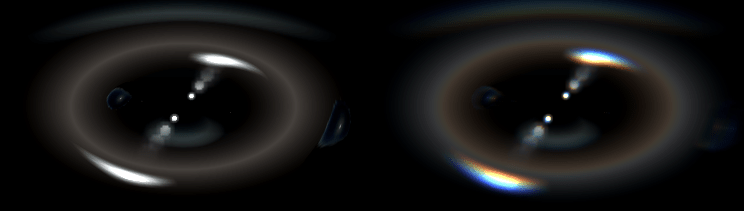``````fixed4 frag (v2f i) : SV_Target
{
//计算失真位置
float2 coords = 2.0 * i.uv - 1.0;
float2 end = i.uv - coords * _ChromaticAberration_Amount;

//uv差值
float2 diff = end - i.uv;
//失真采样像素数
int samples = clamp(int(length(_MainTex_TexelSize.zw * diff / 2.0)), 3, 16);
//每个像素的uv差
float2 delta = diff / samples;
float2 pos = i.uv;
half3 sum = (0.0).xxx, filterSum = (0.0).xxx;

//循环采样计算失真像素点的uv和色值
for (int i = 0; i < samples; i++)
{
half t = (i + 0.5) / samples;
half3 s = tex2Dlod(_MainTex, float4(pos, 0, 0)).rgb;
half3 filter = tex2Dlod(_ChromaticAberration_Spectrum, float4(t, 0, 0, 0)).rgb;

sum += s * filter;
filterSum += filter;
pos += delta;
}

return float4(sum / filterSum, 1);
}
``````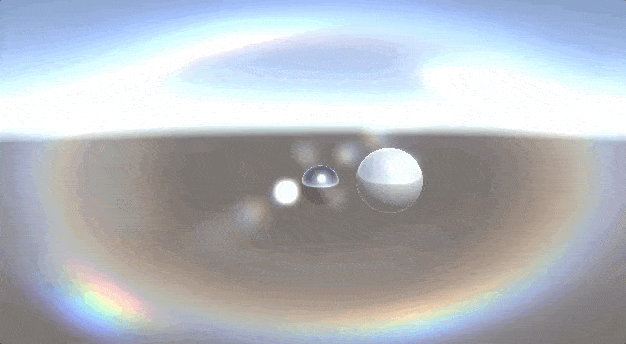## 小结

【博物纳新】是UWA旨在为开发者推荐新颖、易用、有趣的开源项目，帮助大家在项目研发之余发现世界上的热门项目、前沿技术或者令人惊叹的视觉效果，并探索将其应用到自己项目的可行性。很多时候，我们并不知道自己想要什么，直到某一天我们遇到了它。